随时恭候商谈变压器、线圈、空芯线圈等产品相关事宜。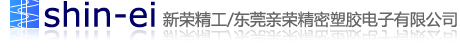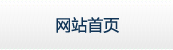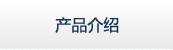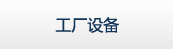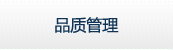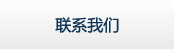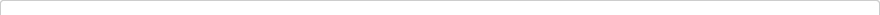### 空心线圈教你如何对线圈进行计算

D------线圈直径
N------线圈匝数
d-----线径
H----线圈高度
W----线圈宽度

l=(0.01*D*N*N)/(L/D+0.44)

l=25330.3/[(f0*f0)*c]

1。针对环形线圈,有以下公式可利用： (铁芯)
L=N2．AL L= 电感值(H)
H-DC=0.4πNI / l N= 线圈匝数（圈）
AL= 感应系数
H-DC=直流磁化力 I= 通过电流(A)
l= 磁路长度(cm)
l及AL值大小，可参照Microl对照表。例如： 以T50-52材，线圈5圈半，其L值为T50-52（表示OD为0.5英吋），经查表其AL值约为33nH
L=33．(5.5)2=998.25nH≒1μH

H-DC=0.4πNI / l = 0.4×3.14×5.5×10 / 3.74 = 18.47 （查表后）

2。介绍一个经验公式
L=(k*μ0*μs*N2*S)/l

μ0 为真空磁导率=4π*10(-7)。(10的负七次方）
μs 为线圈内部磁芯的相对磁导率，空心线圈时μs=1
N2 为线圈圈数的平方
S 线圈的截面积，单位为平方米
l 线圈的长度， 单位为米
k 系数，取决于线圈的半径(R)与长度(l)的比值。